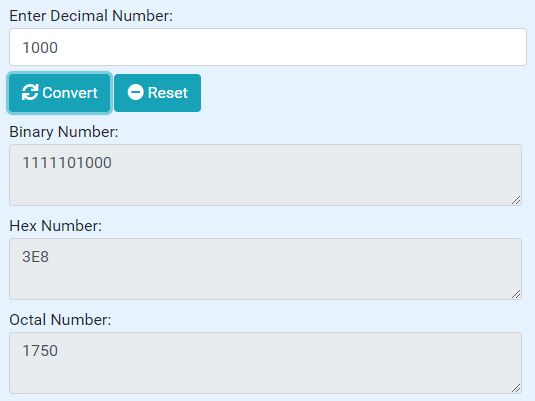# Decimal To Binary/Hex/Octal Converter - jQuery Decimal Conversion

File Size: 4.32 KB 1195 02/15/2019 02:24:00 UTC 02/13/2019 03:11:35 UTC Go to website MITA jQuery based decimal conversion tool which helps you convert decimal number into Binary, Hexadecimal, and Octal numbers with a single click.

## Converting from Decimal to Binary:

• Divide the number by 2.
• Get the integer quotient for the next iteration.
• Get the remainder for the binary digit.
• Repeat the steps until the quotient is equal to 0.

## Converting from Decimal to Hex:

• Divide the decimal number by 16. Treat the division as an integer division.
• Write down the remainder (in hexadecimal).
• Divide the result again by 16. Treat the division as an integer division.
• Repeat step 2 and 3 until result is 0.

## Converting from Decimal to Octal:

• Take the given decimal number
• If the number is less than 8 the octal number is the same
• If the number is greater than 7 then Divide the number with 8
• Note the remainder
• Carry on the step 3 and 4 with the qoutient till it is less than 8
• Write the remainders in reverse order(bottom to top)

How to use it:

1. Create the HTML for the decimal converter.

```<form>

<div>
<div>Enter Decimal Number:</div>
<input type="number" name="decimalInput">
</div>

<div>
<button type="button" name="submitBtn">Convert</button>
<button type="button" name="resetBtn">Reset</button>
</div>

<div>
<div>Binary Number:</div>
<textarea name="binaryArea"disabled></textarea>
</div>

<div>
<div>Hex Number:</div>
<textarea name="hexArea" disabled></textarea>
</div>

<div>
<div>Octal Number:</div>
<textarea name="octalArea" disabled></textarea>
</div>

</form>
```

2. Insert jQuery library and the decimal converter's JavaScript into the web page. Done.

```<script src="https://code.jquery.com/jquery-3.3.1.min.js" integrity="sha384-tsQFqpEReu7ZLhBV2VZlAu7zcOV+rXbYlF2cqB8txI/8aZajjp4Bqd+V6D5IgvKT" crossorigin="anonymous"></script>
<script type="text/javascript" src="assets/js/converter.js"></script>
```

This awesome jQuery plugin is developed by kelleyoj. For more Advanced Usages, please check the demo page or visit the official website.